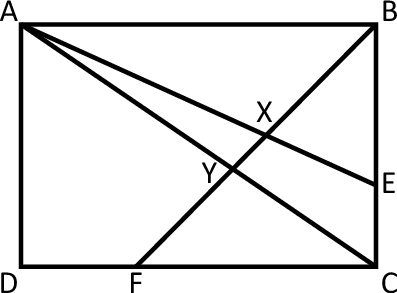Classic Geometry Problem

Geometry Level 5In the figure shown, $ABCD$ is an arbitrary rectangle. Point $E$ lies on side $BC$ such that $\frac{BE}{EC}=2$ and point $F$ lies on $CD$ such that $\frac{CF}{FD}=2$. Segments $AE$ and $AC$ intersect $FB$ at points $X$ and $Y$, respectively. The ratio $FY:YX:XB$ can be expressed as $a:b:c$ where $a$, $b$, and $c$ are coprime, positive integers. Find $a+b+c$.

×